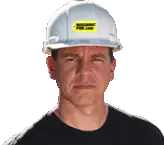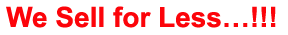# Alternating Current Generators

## Quiz Questions

 1. Most of the electrical power used aboard Navy ships and aircraft as well as in civilian applications is ac dc 2. Ac generators are called Alternators Compressors 3. AC generators vary greatly in size depending upon the load to which they supply power. For example, the alternators in use at hydroelectric plants, such as Hoover Dam, are tremendous in size, generating thousands of kilowatts at very high voltage levels. Another example is the alternator in a typical automobile, which is very small by comparison. It weighs only a few pounds and produces between 100 and 200 watts of power, usually at a potential of 12 volts. True False 4. Regardless of size, all electrical generators, whether dc or ac, depend upon the principle of magnetic induction. An emf is induced in a coil as a result of (1) a coil cutting through a magnetic field, or (2) a magnetic field cutting through a coil. As long as there is relative motion between a conductor and a magnetic field, a voltage will be induced in the conductor. That part of a generator that produces the magnetic field is called the field. That part in which the voltage is induced is called the armature. For relative motion to take place between the conductor and the magnetic field, all generators must have two mechanical parts – a rotor and a stator. The ROTor is the part that ROTates; the STATor is the part that remains STATionary. In a dc generator, the armature is always the rotor. In alternators, the armature may be Rotor Stator either the rotor or stator 5. The rotating-armature alternator is similar in construction to the dc generator (figure 3-1) in that the Armature rotates in a stationary magnetic field Magnetic field rotates around a fixed armature 6. The stators of all rotating-field alternators are about the same. The stator consists of a laminated iron core with the armature windings embedded in this core as shown in figure 3-2. The core is secured to the Stator frame Rotor frame 7. All generators, large and small, ac and dc, require a source of mechanical power to turn their rotors. This source of mechanical energy is called a prime mover. True False 8. A generator that produces a single, continuously alternating voltage is known as a SINGLE-PHASE alternator DOUBLE-PHASE alternator. 9. The output frequency of alternator voltage depends upon the speed of rotation of the rotor and the number of poles. The faster the speed, the higher the frequency. The lower the speed, the lower the frequency. The more poles there are on the rotor, the higher the frequency is for a given speed. When a rotor has rotated through an angle such that two adjacent rotor poles (a north and a south pole) have passed one winding, the voltage induced in that winding will have varied through one complete cycle. For a given frequency, the more pairs of poles there are, the lower the speed of rotation. This principle is illustrated in figure 3-12; a two-pole generator must rotate at four times the speed of an eight-pole generator to produce the same frequency of generated voltage. The frequency of any ac generator in hertz (Hz), which is the number of cycles per second, is related to the number of poles and the speed of rotation, as expressed by the equation F= NP/120 where P is the number of poles, N is the speed of rotation in revolutions per minute (rpm), and 120 is a constant to allow for the conversion of minutes to seconds and from poles to pairs of poles. For example, a 2-pole, 3600-rpm alternator has a frequency of 60 Hz 90 Hz 120 Hz 10. In an alternator, an alternating voltage is induced in the armature windings when magnetic fields of alternating polarity are passed across these windings. The amount of voltage induced in the windings depends mainly on the number of conductors in series per winding, The speed (alternator rpm) at which the magnetic field cuts the winding, and the strength of the magnetic field. All of the above Any of these factors could be used to control the amount of voltage induced in the alternator windings.